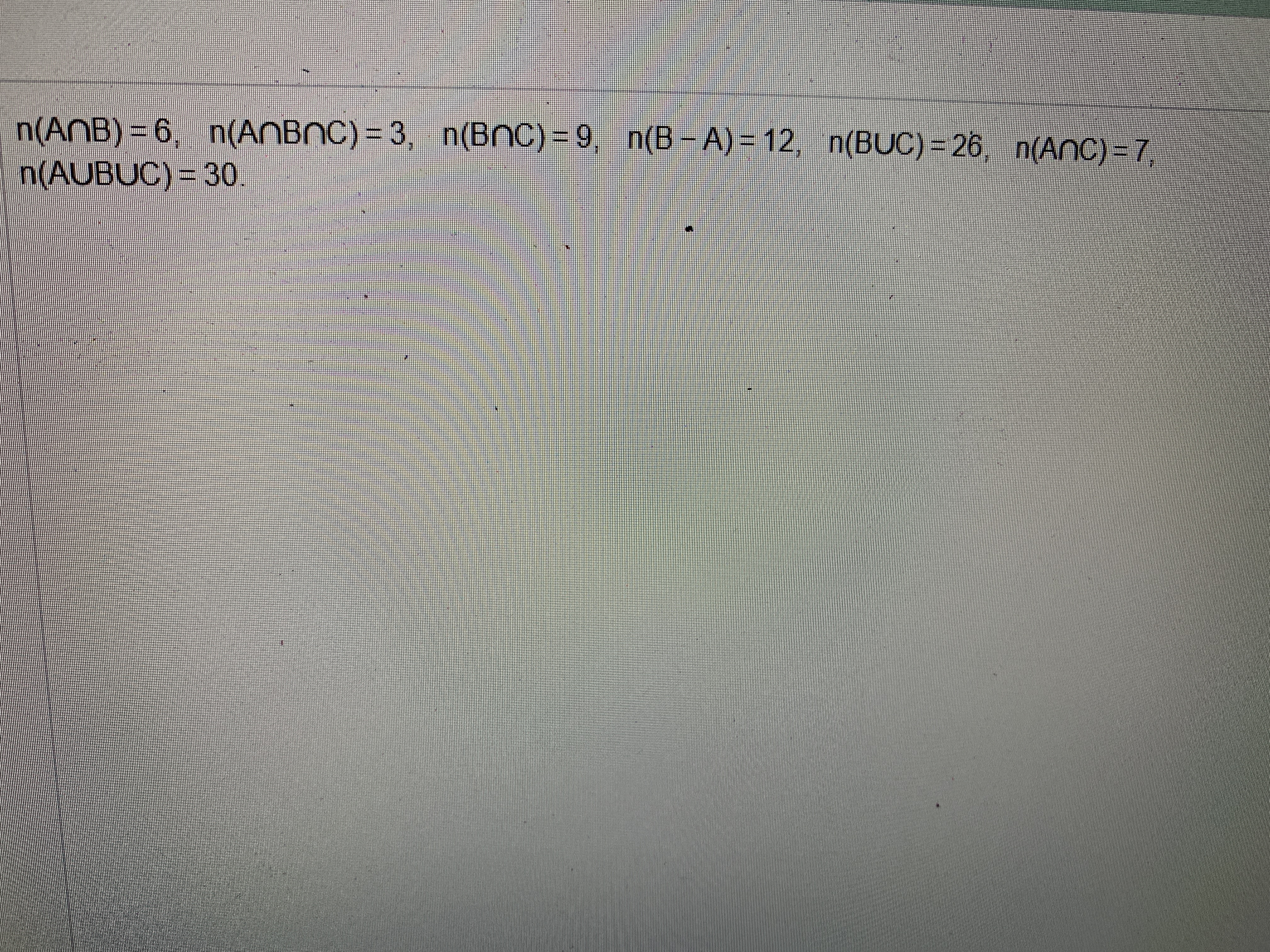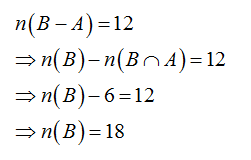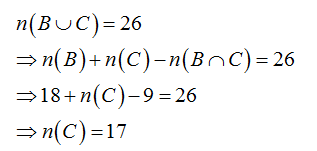# n(ANB) = 6, n(ANBNC) = 3, n(BnC)= 9, n(B - A) = 12, n(BUC) = 26, n(AnC) - 7n(AUBUC)= 30%3D

Question
1 views

Find,if possible,the number of elements in set A,B, and C using the given information.help_outlineImage Transcriptionclosen(ANB) = 6, n(ANBNC) = 3, n(BnC)= 9, n(B - A) = 12, n(BUC) = 26, n(AnC) - 7 n(AUBUC)= 30 %3D fullscreen
check_circle

Consider,Now consider,...

### Want to see the full answer?

See Solution

#### Want to see this answer and more?

Solutions are written by subject experts who are available 24/7. Questions are typically answered within 1 hour.*

See Solution
*Response times may vary by subject and question.
Tagged in